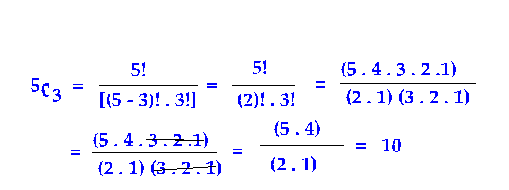## Binomial Coefficient

#### 4. Binomial Coefficients:

nC0, nC1, nC2, nC3,….. nCrnCn are called Binomial Coefficients.

Recall the formula for factorial of a number n defined as

n! = n (n – 1) (n - 2 ) (n - 3) (n - 4)……3.2.1

For Eg,
5! = 5.4.3.2.1 = 120
4! = 4.3.2.1 = 24
3! = 3.2.1 = 6
2! = 2.1= 2
1! = 1 and note that
0! = 1

The values of the Binomial Coefficients are determined by the following formula:Consider the Examples :